# Math Antics - Common Denominator ECD

##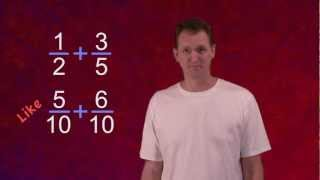By mathantics# Fraction to Percent | MathHelp.com

##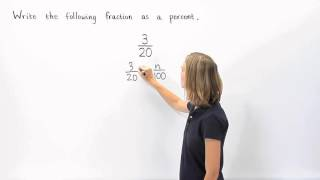By MathHelp.com# Math Antics - Working With Parts

##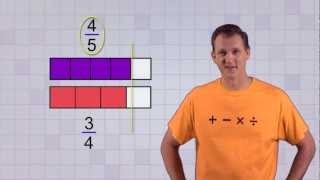By mathantics# Understand & Calculate Equivalent Fractions - 

##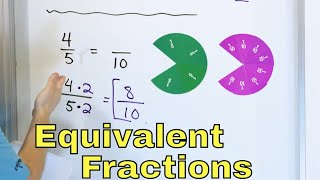By Math and Science# Understand & Calculate Equivalent Fractions - 

##By Math and Science

Quality Math And Science Videos that feature step-by-step example problems!# Lesson 1 - Multiply Whole Numbers By Fractions (5th Grade Math)

##By Lumos Learning

This is just a few minutes of a complete course. Get all lessons & more subjects at: http://www.MathTutorDVD.com​. In this lesson the student will learn how to multiply a whole number by a fraction and simplify the result.# Surface Area and Volume of a Sphere | MathHelp.com

##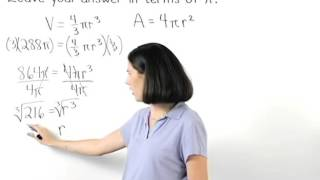By MathHelp.com# Partial Fraction Decomposion

##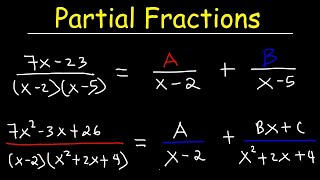By The Organic Chemistry Tutor

This precalculus video tutorial provides a basic introduction into partial fraction decomposition. The full version of this video contains plenty of examples and practice problems with repeated linear factors and repeated quadratic factors. Partial fraction decomposition is the process of taking a complex fraction and breaking it into multiple simpler fractions. It's the reverse of adding combining two fractions into a single fraction.# Rational Equations | MathHelp.com

##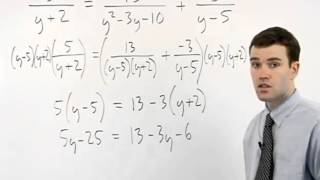By MathHelp.com# 20 - The Rational Root Theorem, Part 1 (Rational Roots of Polynomials)

##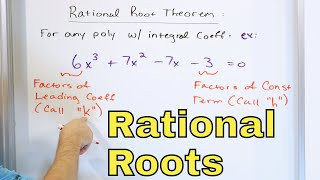By Math and Science

Quality Math And Science Videos that feature step-by-step example problems!# Percent Proportion

##By Anywhere Math# Integration By Partial Fractions

##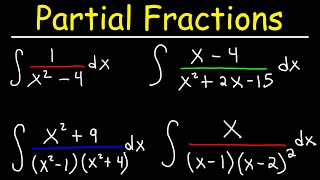By The Organic Chemistry Tutor

This calculus video tutorial provides a basic introduction into integrating rational functions using the partial fraction decomposition method. Partial fraction decomposition is the process of breaking a single complex fraction into multiple simpler fractions. The integrals of many rational functions lead to a natural log function with absolute value expressions. This video explains what to do when you have repeated linear factors and quadratic factors. This tutorial contains many examples and practice problems on integration by partial fractions.# Math Antics - Proportions

##By mathantics# Volume of Rectangular Prisms

##By Anywhere Math# 15 - Complex Numbers & the Complex Plane

##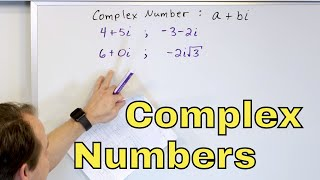By Math and Science

Quality Math And Science Videos that feature step-by-step example problems!# Math Antics - Number Patterns

##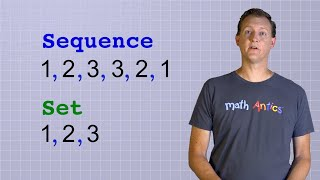By mathantics##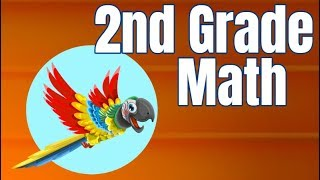By

2nd Grade math is fun with these learning videos! Each lesson can help students with word problems, math games or help prepare for a test! Great for a review of 2nd grade or for an introduction!# Ratios Introduction - what are ratios?

##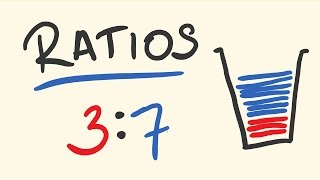By tecmath# Multiplying Fractions

##By Anywhere Math# 06 - Review of Essential Trigonometry (Sin, Cos, Tangent - Trig Identities & Functions)

##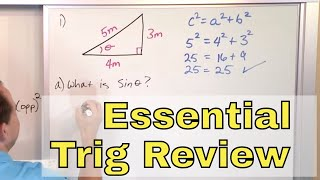By Math and Science

Quality Math And Science Videos that feature step-by-step example problems!